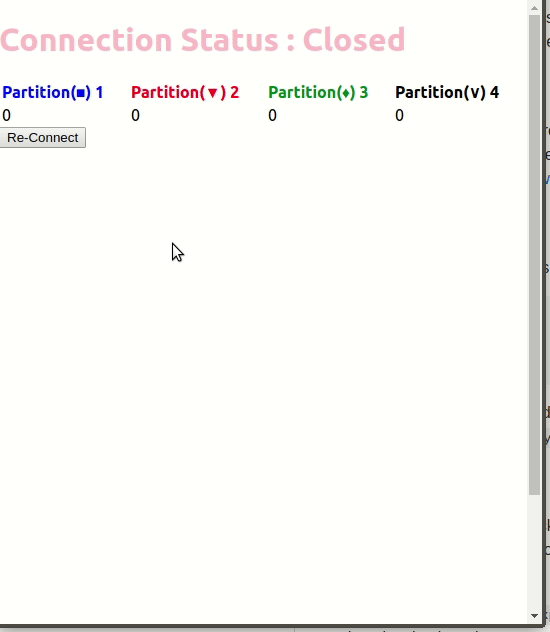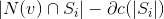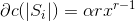{{ message }}

Streaming Graph Server with partitioning

# tmkasun/streaming_graph_partitioning

Switch branches/tags
Nothing to show

## Files

Failed to load latest commit information.
Type
Name
Commit time

# Streaming Graph partitioning

This repository contains the slightly modified version of Fennel, and Linear Deterministic Greedy (LDG) partitioning algorithms to work with stream of edges (a pair of vertices). The only difference is , Practitioner accept edge of a graph instead of single vertexes and add both vertexes(aka Nodes) to partitions.

I have develp this code for testing out different streaming graph partitioning algorithms, and validate and try out the partioninig quality with visualization.## Technology stack

Partitioning algorithms are implemented in cpp+11 and visualization is done using Cytoscape.js. Use Apache Kafka for consume stream of edges in a graph. A python script is used for streaming edges from a graph in a file. It can also stream a graph by taking user inputs from the terminal. Practitioner accept the graph as a stream of edges , where edge contains a space separated vertex pair, i:e `22 45`

## More on Streaming Graphs

This was implemented as a part of adding new streaming graph partitioning algorithm to Jasmine Graph server. You could try out Jasmine graph server for more robust work on graph partitioning and analysis.

## Algorithms

### Implementation of Fennel

I have used following maximization formulae for deriving the partition index for most optimum partitioning. The hubristic used in here is, if a vertex having more neighbors and have not reached to it's maximum capacity, Then it(Partition) will have most likelihood to accept that vertex.

Inter partition costIntra partition cost## Results comparison

### LDG

`````` ***Received the end of stream
0 => Vertext count = 2237
0 => Edges count = 1125
0 => Edge cuts count = 440
0 => Cut ratio = 0.28115
1 => Vertext count = 2235
1 => Edges count = 1162
1 => Edge cuts count = 430
1 => Cut ratio = 0.270101
2 => Vertext count = 2236
2 => Edges count = 1156
2 => Edge cuts count = 447
2 => Cut ratio = 0.278852
3 => Vertext count = 2236
3 => Edges count = 1153
3 => Edge cuts count = 455
3 => Cut ratio = 0.28296
Time taken = 3.88941e+07 micro seconds
``````

### Fennel

`````` ***Received the end of stream
0 => Vertext count = 1711
0 => Edges count = 688
0 => Edge cuts count = 1758
0 => Cut ratio = 0.718724
1 => Vertext count = 1711
1 => Edges count = 580
1 => Edge cuts count = 1900
1 => Cut ratio = 0.766129
2 => Vertext count = 1710
2 => Edges count = 553
2 => Edge cuts count = 1973
2 => Cut ratio = 0.781077
3 => Vertext count = 1711
3 => Edges count = 545
3 => Edge cuts count = 1961
3 => Cut ratio = 0.782522
Time taken = 5.8881e+07 micro seconds
``````

### Hash

`````` ***Received the end of stream
0 => Vertext count = 1644
0 => Edges count = 323
0 => Edge cuts count = 2601
0 => Cut ratio = 0.889535
1 => Vertext count = 1695
1 => Edges count = 310
1 => Edge cuts count = 2644
1 => Cut ratio = 0.895058
2 => Vertext count = 1672
2 => Edges count = 306
2 => Edge cuts count = 2648
2 => Cut ratio = 0.896412
3 => Vertext count = 1646
3 => Edges count = 326
3 => Edge cuts count = 2535
3 => Cut ratio = 0.886054
Time taken = 428249 micro seconds
``````

## Sample output

`````` ***Received the end of stream
0 => Vertext count = 5
0 => Edges count = 4
0 => Edge cuts count = 4
0 => Cut ratio = 0.5
Printing edge cuts of 0 partition
8 ____
| ---> 9

39 ____
| ---> 36

14 ____
| ---> 17

35 ____
| ---> 34

Printing edge list of 0 partition
6 ____
| ===> 8

7 ____
| ===> 8

14 ____
| ===> 16
| ===> 29

1 => Vertext count = 5
1 => Edges count = 3
1 => Edge cuts count = 6
1 => Cut ratio = 0.666667
Printing edge cuts of 1 partition
9 ____
| ---> 8

36 ____
| ---> 39

19 ____
| ---> 20

36 ____
| ---> 37

14 ____
| ---> 13

36 ____
| ---> 38

Printing edge list of 1 partition
18 ____
| ===> 19

35 ____
| ===> 36

41 ____
| ===> 42

2 => Vertext count = 4
2 => Edges count = 1
2 => Edge cuts count = 3
2 => Cut ratio = 0.75
Printing edge cuts of 2 partition
20 ____
| ---> 19

37 ____
| ---> 36

15 ____
| ---> 13

Printing edge list of 2 partition
9 ____
| ===> 10

3 => Vertext count = 4.5
3 => Edges count = 3
3 => Edge cuts count = 5
3 => Cut ratio = 0.625
Printing edge cuts of 3 partition
17 ____
| ---> 14

34 ____
| ---> 35

13 ____
| ---> 14

38 ____
| ---> 36

13 ____
| ---> 15

Printing edge list of 3 partition
5 ____
| ===> 13

12 ____
| ===> 13

33 ____
| ===> 34

Time taken = 28433 micro seconds
``````

Streaming Graph Server with partitioning

## Releases

No releases published

## Packages 0

No packages published